MORE IN Elements of Civil Engg. & Engg. Mechanics
VTU First Year Engineering (P Cycle) (Semester 1)
Elements of Civil Engg. & Engg. Mechanics
December 2014
Total marks: --
Total time: --
INSTRUCTIONS
(1) Assume appropriate data and state your reasons
(2) Marks are given to the right of every question
(3) Draw neat diagrams wherever necessary

1 (a) Briefly explain the role of civil engineers in the infrastructural development.
10 M
1 (b) In the traigle ABC, a force at 'A' produces a clockwise moment of 90 KN-m at B and an anticlockwise moment of 45 kN-m at C. Find the magnitude and direction of the force.6 M
1 (c) Define force and its characteristics.
4 M

2 (a) Explain the following with neat sketches:
i) Principle of superposition of forces
ii) Principle of transmissibility of forces.
iii) Couple and its characteristics.
10 M
2 (b) Draw typical cross section of a road and explain the parts.
10 M

3 (c) State and prove parallelgram law of forces.
6 M
3 (a) Four co-planar forces acting at a point are shown in Fig. Q3(a). One of the forces is unknown and its magnitude is shown by 'p'. The resultant has a magnitude of 500 N and is acting along the x-axis. Determine the unknown force 'P' and its inclination with x-axis.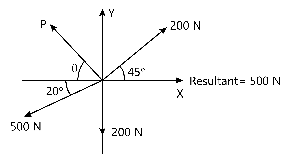8 M
3 (b) State and prove Varignons theorem of moments.
6 M

4 (a) Determine the magnitude, direction of the resultant force for the force system as shown in Fig. Q4(a). Locate the resultant force with respect to point D.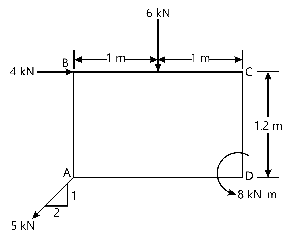8 M
4 (b) 26 kN force is the resultant of the two forces, one of which is as shown in Fig, Q4 (b). Determine the other force.8 M
4 (c) Explain the principle of resolved parts.
4 M

5 (a) Determine the reactions at constant points for spheres A, B and C as shown in Fig. Q5 (a). it is given that WA-WB=4 kN, WC-6kN, dA=dD=500mm, dc=800mm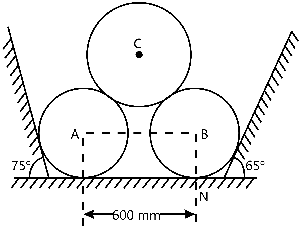12 M
5 (b) For the beam with loading shown in Fig. Q5(b). determine the reactions at the supports.8 M

6 (a) State and prove Lamis theorem.
8 M
6 (b) The ladder shown in Fig. Q6 (b) is 4 m long and is supported by a horizontal floor and vertical wall. The co-efficient of friction at the wall is 0.25 and at the floor is 0.50. The weight of the ladder is 200 N, considered concentrated at 'G'. The ladder supports a vertical load of 1000 N at 'C'. Determine the ractions 'A' and 'B' and compute the least value of '?' at which the ladder may be placed without slipping.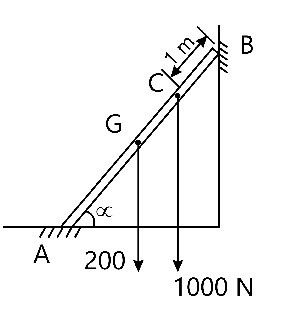8 M
6 (c) State laws of friction.
4 M

7 (a) Determine the centroid of a semi-circular lamina of radius R by method of integration.
8 M
7 (b) Determine the moment of inertia of the section shown in Fig. Q7(b) about its centroidal axes. Calculated the least radius of gyration for the section as well.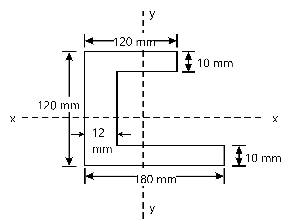12 M

8 (a) State and prove parallel axis theorem.
6 M
8 (b) Locate the centroid of the shaded area as shown in Fig. Q8 (b)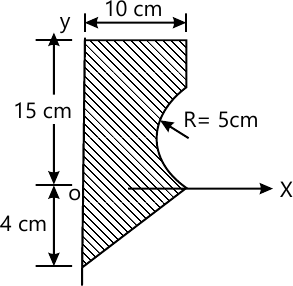>

8 M
8 (c) Derive an expression for moment of inertia of a triangle with respect to horizontal centroidal axis.
6 M

9 (a) What is centrifugal force? What is super elevation?
4 M
9 (b) Determine the position at which the ball it thrown up the plane will strike the inclined plane as shown in Fig. Q9(b). The initial velocity is 30 m/s and angle of projection is $\tan^{-1} \left( \dfrac {4}{3} \right)$ with horizontal.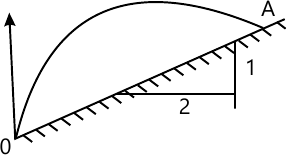8 M
9 (c) A stone is dropped from the top of the tower 50 m high. At the same time another stone is thrown up from the tower with a velocity of 25 m/s, At what distance from the top and after how much time the two stones cross each other?
8 M

10 (a) What is projectile? Define the following terms briefly: i) Angle of projection
ii) Horizontal range
iii) Vertical height
iv) Time of flight
10 M
10 (b) A burglar's car starts at an acceleration of 2 m/s2. A police vigilant party came after 5 s and continued to chase the burglar's car with a uniform velocity of 20 m/s. Find the time taken in which the police van will overtake the car.
10 M

More question papers from Elements of Civil Engg. & Engg. Mechanics